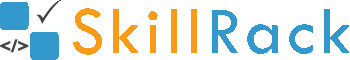Must Solve 100 Programs for Campus Placement Part - 5/104 months ago

1) Length of the String

The program must accept a string S as the input. The program must print the length of S as the output.

Boundary Condition(s):
1 <= Length of S <= 1000

Example Input/Output 1:
Input:
hello

Output:
5

Example Input/Output 2:
Input:
pineapple

Output:
9

Click the link below to solve this program
http://www.skillrack.com/solve/7936

2) Middle Three Characters

A string S of length 7 is passed as the input to the program. The program must print the middle three characters as the output.

Example Input/Output:
Input:
brinjal

Output
inj

Click the link below to solve this program
http://www.skillrack.com/solve/8088

3) Print Last Four Digits

The program must accept an integer N as the input. The program must print the last four digits in N as the output.

Example Input/Output 1:
Input:
23847

Output:
3847

Example Input/Output 2:
Input:
23477234

Output:
7234

Click the link below to solve this program
http://www.skillrack.com/solve/8057

4) Discount - 20%

The program must accept the price of an item as the input. The program must print the price of the item after 20 percent discount with the precision up to two decimal places as the output.

Example Input/Output 1:
Input:
29

Output:
23.20

Click the link below to solve this program
http://www.skillrack.com/solve/8089

5) Odd Even Mixed

Accept two integers A and B as the input.
- If A and B are even then print "EVEN" as the output.
- If A and B are odd then print "ODD" as the output.
- If A is odd and B is even or if A is even and B is odd then print "MIXED" as the output.

Example Input/Output 1:
Input:
22 14

Output:
EVEN

Example Input/Output 2:
Input:
11 47

Output:
ODD

Example Input/Output 3:
Input:
34 97

Output:
MIXED

Click the link below to solve this program
http://www.skillrack.com/solve/8090

6) Sum of Two to Get Third Integer

The program must accept three integers as the input. The program must print yes if any two of the three integers can be added to obtain the remaining integer as the output. Else the program must print no as the output.

Example Input/Output 1:
Input:
-1 2 3

Output
yes

Explanation:
-1 + 3 = 2

Click the link below to solve this program
http://www.skillrack.com/solve/8092

7) N Integers From N

The program must accept an integer N as the input. Then the program must print N integers from N as the output.

Example Input/Output 1:
Input:
5

Output:
5 6 7 8 9

Click the link below to solve this program
http://www.skillrack.com/solve/8094

8) Calculate Park Entrance Fee

In a park, they charge X rupees for adults and Y rupees for kids for entry. The program must accept two integers A and B as the input(where A is the number of adults and B is the number of kids). The program must print the total amount charged as the output.

Example Input/Output 1:
Input:
100 50
2 3

Output:
350

Example Input/Output 2:
Input:
30 10
4 5

Output:
170

Click the link below to solve this program
http://www.skillrack.com/solve/8101

9) Multiple of 30 Plus 1 or 2

The program must accept an integer N as the intput. The program must print YES if N is exactly one or two more than a multiple of 30 as the output. Else the program must print NO as the output.

Example Input/Output 1:
Input:
31

Output:
YES

Example Input/Output 2:
Input:
63

Output:
NO

Click the link below to solve this program
http://www.skillrack.com/solve/8093

10) Check if Multiple of N1 and N2

The program must accept three integers N1, N2 and N3 as the input. The program must print yes if N2 is a multiple of N1 and N3 is a multiple of N2. Else the program must print no as the output.

Example Input/Output 1:
Input:
8 32 64

Output:
yes

Click the link below to solve this program
http://www.skillrack.com/solve/8099